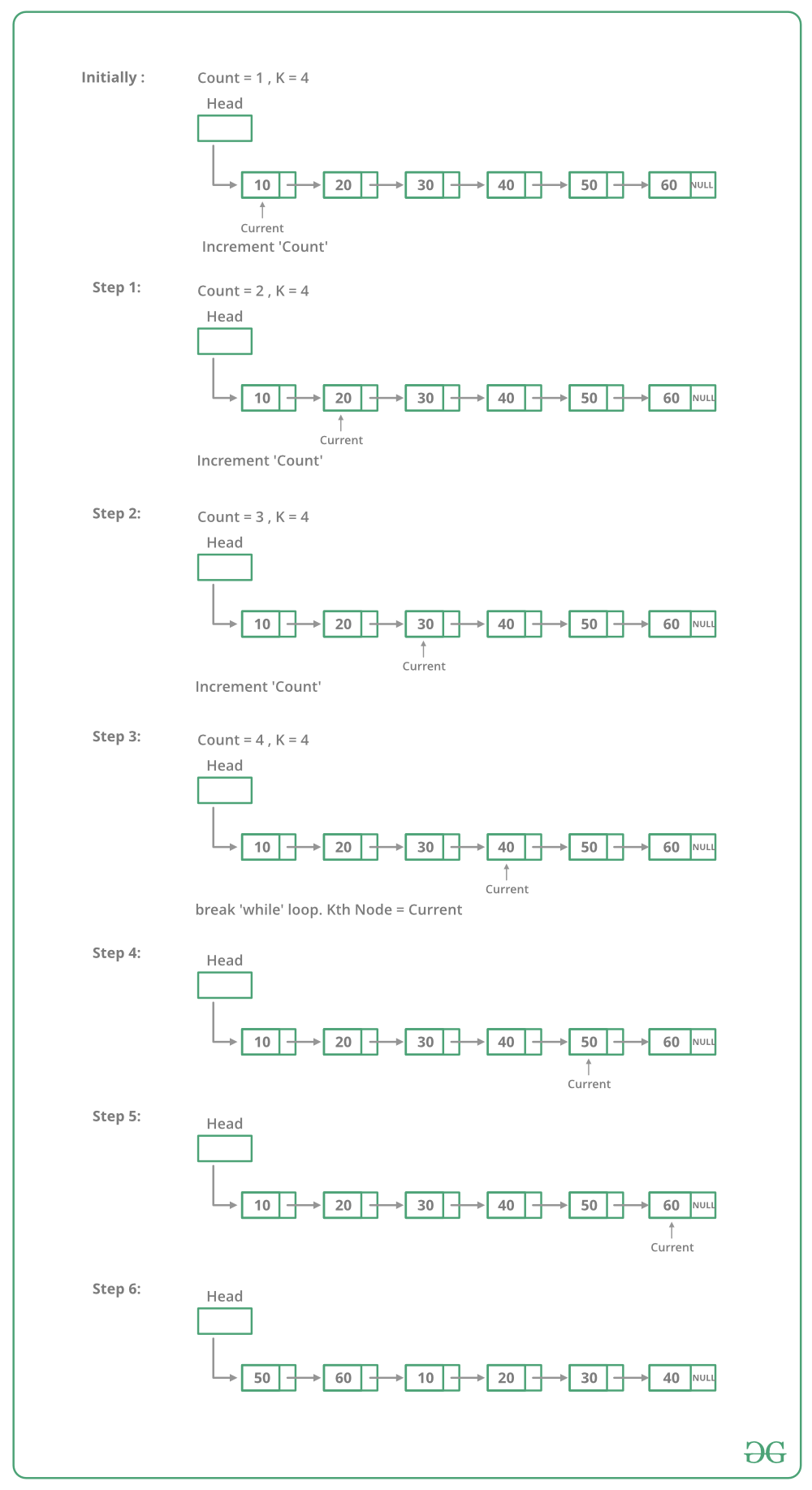# C++ Program For Rotating A Linked List

• Last Updated : 21 Dec, 2021

Given a singly linked list, rotate the linked list counter-clockwise by k nodes. Where k is a given positive integer. For example, if the given linked list is 10->20->30->40->50->60 and k is 4, the list should be modified to 50->60->10->20->30->40. Assume that k is smaller than the count of nodes in a linked list.

Method 1:
To rotate the linked list, we need to change the next of kth node to NULL, the next of the last node to the previous head node, and finally, change the head to (k+1)th node. So we need to get hold of three nodes: kth node, (k+1)th node, and last node.
Traverse the list from the beginning and stop at kth node. Store pointer to kth node. We can get (k+1)th node using kthNode->next. Keep traversing till the end and store a pointer to the last node also. Finally, change pointers as stated above.

Below image shows how to rotate function works in the code :## C++

 `// C++ program to rotate a ``// linked list counter clock wise``#include ``using` `namespace` `std;`` ` `// Link list node ``class` `Node ``{``    ``public``:``    ``int` `data;``    ``Node* next;``};`` ` `// This function rotates a linked list``// counter-clockwise and updates the``// head. The function assumes that k is``// smaller than size of linked list.``// It doesn't modify the list if``// k is greater than or equal to size``void` `rotate(Node** head_ref, ``int` `k)``{``    ``if` `(k == 0)``        ``return``;`` ` `    ``// Let us understand the below``    ``// code for example k = 4 and``    ``// list = 10->20->30->40->50->60.``    ``Node* current = *head_ref;`` ` `    ``// current will either point to``    ``// kth or NULL after this loop.``    ``// current will point to node``    ``// 40 in the above example``    ``int` `count = 1;``    ``while` `(count < k && ``           ``current != NULL) ``    ``{``        ``current = current->next;``        ``count++;``    ``}`` ` `    ``// If current is NULL, k is greater ``    ``// than or equal to count of nodes ``    ``// in linked list. Don't change the ``    ``// list in this case``    ``if` `(current == NULL)``        ``return``;`` ` `    ``// current points to kth node.``    ``// Store it in a variable. kthNode``    ``// points to node 40 in the above ``    ``// example``    ``Node* kthNode = current;`` ` `    ``// current will point to``    ``// last node after this loop``    ``// current will point to``    ``// node 60 in the above example``    ``while` `(current->next != NULL)``        ``current = current->next;`` ` `    ``// Change next of last node to ``    ``// previous head. Next of 60 is ``    ``// now changed to node 10``    ``current->next = *head_ref;`` ` `    ``// Change head to (k+1)th node``    ``// head is now changed to node 50``    ``*head_ref = kthNode->next;`` ` `    ``// Change next of kth node to NULL``    ``// next of 40 is now NULL``    ``kthNode->next = NULL;``}`` ` `// UTILITY FUNCTIONS ``// Function to push a node ``void` `push(Node** head_ref, ``          ``int` `new_data)``{``    ``// Allocate node ``    ``Node* new_node = ``new` `Node();`` ` `    ``// Put in the data ``    ``new_node->data = new_data;`` ` `    ``// Link the old list off the ``    ``// new node ``    ``new_node->next = (*head_ref);`` ` `    ``// Move the head to point to the ``    ``// new node ``    ``(*head_ref) = new_node;``}`` ` `// Function to print linked list ``void` `printList(Node* node)``{``    ``while` `(node != NULL) ``    ``{``        ``cout << node->data << ``" "``;``        ``node = node->next;``    ``}``}`` ` `// Driver code``int` `main(``void``)``{``    ``// Start with the empty list ``    ``Node* head = NULL;`` ` `    ``// Create a list ``    ``// 10->20->30->40->50->60``    ``for` `(``int` `i = 60; i > 0; i -= 10)``        ``push(&head, i);`` ` `    ``cout << ``"Given linked list "``;``    ``printList(head);``    ``rotate(&head, 4);`` ` `    ``cout << ``"Rotated Linked list "``;``    ``printList(head);`` ` `    ``return` `(0);``}``// This code is contributed by rathbhupendra`

Output:

```Given linked list
10  20  30  40  50  60
50  60  10  20  30  40```

Time Complexity: O(n) where n is the number of nodes in Linked List. The code traverses the linked list only once.

Method 2:
To rotate a linked list by k, we can first make the linked list circular and then moving k-1 steps forward from head node, making (k-1)th node’s next to null and make kth node as head.

## C++

 `// C++ program to rotate a ``// linked list counter clock wise``#include ``using` `namespace` `std;`` ` `// Link list node ``class` `Node ``{``    ``public``:``    ``int` `data;``    ``Node* next;``};`` ` `// This function rotates a linked list``// counter-clockwise and updates the``// head. The function assumes that k is``// smaller than size of linked list.``void` `rotate(Node** head_ref, ``int` `k)``{``    ``if` `(k == 0)``        ``return``;`` ` `    ``// Let us understand the below``    ``// code for example k = 4 and``    ``// list = 10->20->30->40->50->60.``    ``Node* current = *head_ref;`` ` `    ``// Traverse till the end.``    ``while` `(current->next != NULL)``        ``current = current->next;`` ` `    ``current->next = *head_ref;``    ``current = *head_ref;`` ` `    ``// Traverse the linked list to ``    ``// k-1 position which will be ``    ``// last element for rotated array.``    ``for` `(``int` `i = 0; i < k - 1; i++)``        ``current = current->next;`` ` `    ``// Update the head_ref and last ``    ``// element pointer to NULL``    ``*head_ref = current->next;``    ``current->next = NULL;``}`` ` `// UTILITY FUNCTIONS ``// Function to push a node ``void` `push(Node** head_ref, ``          ``int` `new_data)``{``    ``// Allocate node ``    ``Node* new_node = ``new` `Node();`` ` `    ``// Put in the data ``    ``new_node->data = new_data;`` ` `    ``// Link the old list off the``    ``// new node ``    ``new_node->next = (*head_ref);`` ` `    ``// Move the head to point to ``    ``// the new node ``    ``(*head_ref) = new_node;``}`` ` `// Function to print linked list ``void` `printList(Node* node)``{``    ``while` `(node != NULL) ``    ``{``        ``cout << node->data << ``" "``;``        ``node = node->next;``    ``}``}`` ` `// Driver code``int` `main(``void``)``{``    ``// Start with the empty list ``    ``Node* head = NULL;`` ` `    ``// Create a list ``    ``// 10->20->30->40->50->60``    ``for` `(``int` `i = 60; i > 0; i -= 10)``        ``push(&head, i);`` ` `    ``cout << ``"Given linked list "``;``    ``printList(head);``    ``rotate(&head, 4);`` ` `    ``cout << ``"Rotated Linked list "``;``    ``printList(head);`` ` `    ``return` `(0);``}``// This code is contributed by pkurada`

Output:

```Given linked list
10 20 30 40 50 60